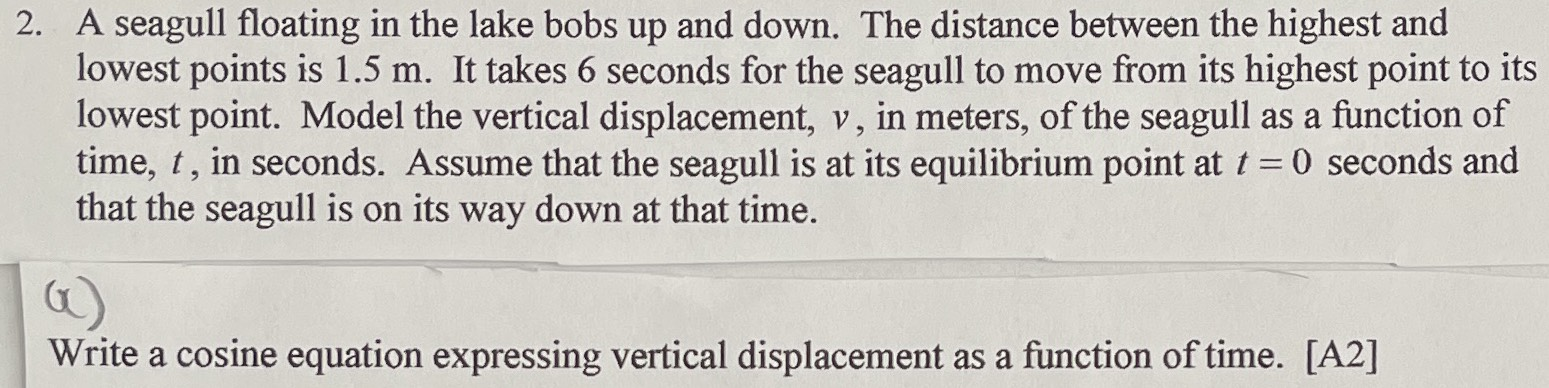### ¿Todavía tienes preguntas de matemáticas?

Pregunte a nuestros tutores expertos
Algebra
Pregunta2. A seagull floating in the lake bobs up and down. The distance between the highest and lowest points is $$1.5 m$$ . It takes $$6$$ seconds for the seagull to move from its highest point to its lowest point. Model the vertical displacement, $$v$$ , in meters, of the seagull as a function of time, $$t$$ , in seconds. Assume that the seagull is at its equilibrium point at $$t = 0$$ seconds and that the seagull is on its way down at that time. Write a cosine equation expressing vertical displacement as a function of time.

$$0.75cos(\frac{\pi }{6} t)+ 0.75$$